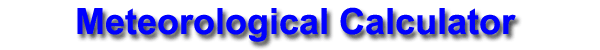Temperature Conversion Enter a number then click on the the conversion button. Fahrenheit (o F) Celsius (o C)

 Wind Speed Calculations Enter a speed into any blank to convert to all other wind speeds. mph   knots     m/sec     km/hr Wind Chill Calculation Enter the temperature in degrees Fahrenheit and wind speed in mph, then click on the Calculate WC to compute the windchill index. Temp (o F) Wind (mph) Wind Chill Index
 Relative Humidity Calculation Enter the air temperature and the dew point in degrees Fahrenheit, then click on the Calculate RH to compute the relative humidity. Temp (o F) Dew Point

 Heat Index Calculations Enter the temperature in degrees Fahrenheit and relative humidity (RH) in percent (without the % sign), or the Dew Point (Tdp).  Then click on the Calculate HI to compute the heat index (HI). Temp (o F) RH HI Tdp OR Temp (o F) Tdp HI RH
 Station Pressure Calculation What is the current altimeter (inches)? What is the station elevation? (feet) i.e. St. Louis - Lambert= 710 The Station Pressure in inches is: The Station Pressure in millibars is: The Pressure Altitude in feet is: